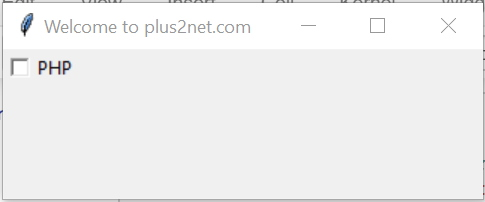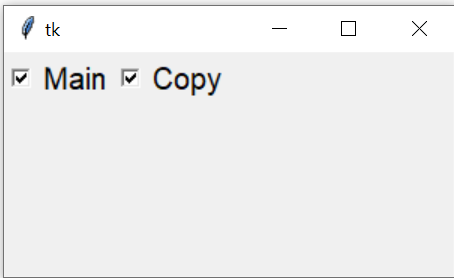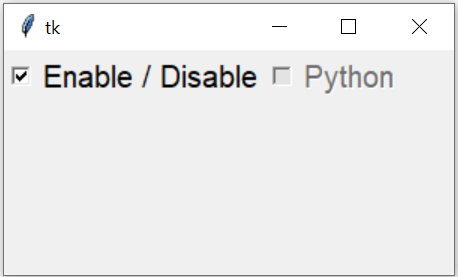# Python tkinter CheckbuttonTkinter Checkbutton reading the Checked or Unchecked value & setting the state to disabled or enable
``````import tkinter as tk
my_w = tk.Tk()
my_w.geometry("500x500")  # Size of the window

c_v1=IntVar()
c1 = tk.Checkbutton(my_w,text='PHP',variable=c_v1,
onvalue=1,offvalue=0)
c1.grid(row=2,column=2)

my_w.mainloop()  # Keep the window open``````
In above code we have linked the IntVar() variable c_v1 to the checkbutton. This value we will be using for our reading and writting ( setting ) the status of the checkbutton.

`onvalue=1` mean the variable c_v1 will get value 1 when checkbutton is clicked. or checked
`offvalue=0` mean the variable c_v1 will get value 0 when checkbutton is unchecked

How to read the status ( get value of the associated variable ) of the check box. ?
``my_val=c1_v1.get() #c1_v1 is the variable connected to checkbutton ``
How to set the value of the checkbutton ( to Check or Uncheck )?
``c1_v1.set(1)``
We will use the above concepts. Note that checkbutton can have values 1 or 0 ( integer variable ), Yes or No ( string variable) , True or False ( Boolean variable ). We will learn all three types here.

## Events of checkbutton

Capturing click event of checkbox and display the value of the variable
``````import tkinter as tk
my_w = tk.Tk()
my_w.geometry("500x500")  # Size of the window

def my_upd():
print('Check box value :',c1_v1.get())

c1_v1=tk.IntVar()
c1 = tk.Checkbutton(my_w, text='PHP',variable=c1_v1,
onvalue=1,offvalue=0,command=my_upd)
c1.grid(row=2,column=2)

my_w.mainloop()  # Keep the window open``````
Capturing click event of checkbox and display the string variable
``````import tkinter as tk
my_w = tk.Tk()
my_w.geometry("500x500")

def my_upd():
print('Check box value :',c1_v1.get())

c1_v1=tk.StringVar()
c1 = tk.Checkbutton(my_w, text='PHP', variable=c1_v1,
onvalue='Yes',offvalue='No',command=my_upd)
c1.grid(row=2,column=2)

my_w.mainloop()``````
How to use Checkbutton to wrap text in Entry widget

Now let us use one Boolean variable
``````import tkinter as tk
my_w = tk.Tk()
my_w.geometry("500x500")

def my_upd():
print('Check box value :',c1_v1.get())

c1_v1=tk.BooleanVar()
c1 = tk.Checkbutton(my_w, text='PHP', variable=c1_v1,
onvalue=True,offvalue=False,command=my_upd)
c1.grid(row=2,column=2)

my_w.mainloop()``````

## How to set a default value ( check or uncheck ) to a checkbutton

``````import tkinter as tk
my_w = tk.Tk()
my_w.geometry("500x500")

def my_upd():
print('Check box value :',c1_v1.get())

c1_v1=tk.BooleanVar()
c1 = tk.Checkbutton(my_w, text='PHP', variable=c1_v1,
onvalue=True,offvalue=False,command=my_upd)
c1.grid(row=2,column=2)
c1_v1.set(False) # Change  to True to check the Checkbutton by default

my_w.mainloop()``````
Using all above knowledge let us try to make second text button check or uncheck based on updating of first checkbox. Here we need to read the data of first checkbutton and then copy the same to second checkbutton. Note that checkbuttons can hold values other than 0 or 1 ( True or False ).
``````import tkinter as tk
my_w = tk.Tk()
my_w.geometry("500x500")

def my_upd():
c2_v2.set(c1_v1.get())
print('Check box value :',c1_v1.get())

c1_v1=tk.StringVar()
c1 = tk.Checkbutton(my_w, text='PHP', variable=c1_v1,
onvalue='Yes',offvalue='No',command=my_upd)
c1.grid(row=2,column=2)
c1_v1.set('Yes')   # Default value is kept as checked

c2_v2=tk.StringVar()
c2 = tk.Checkbutton(my_w, text='MySQL', variable=c2_v2,
onvalue='Yes',offvalue='No')
c2.grid(row=2,column=3)
c2_v2.set('Yes')   # Default value is kept as checked

my_w.mainloop() ``````

## Checkbutton select() deselect() & toggle()`select()` : To check the Checkbutton
`deselect()` : to Uncheck the Checkbutton
`toggle()` : to change the status
``````import tkinter  as tk
my_w = tk.Tk()
my_w.geometry("300x150")
def my_upd():
if(c4_v.get()==1):
c4.deselect()
else:
c4.select()

c1=tk.Checkbutton(my_w,text='Main',command=my_upd,font=18)
c1.grid(row=1,column=1)
c4_v=tk.IntVar(my_w)
c4=tk.Checkbutton(my_w,text='Copy',font=18,variable=c4_v)
c4.grid(row=1,column=2)
my_w.mainloop()``````
The function my_upd can be changed to add toggle()
``````def my_upd():
c4.toggle()``````
We can keep the Main Checkbutton in checked mode by adding this line after declaring c1.
``c1.select()``

## Enable or Disable Checkbutton

By using config we can manage the state option of the Checkbutton.``````c1.config(state='disabled') # c1 is the Checkbutton
c1.config(state='normal')``````
Enable or disable Checkbutton by using a Checkbutton.
``````import tkinter  as tk
my_w = tk.Tk()
my_w.geometry("300x150")
def my_upd():
if(c1_v1.get()=='Yes'):c2.config(state='disabled')
else:c2.config(state='normal')
c1_v1=tk.StringVar(my_w)
c1_v1.set('')
c1=tk.Checkbutton(my_w,text='Enable / Disable',variable=c1_v1,
onvalue='Yes',offvalue='',command=my_upd,font=18)
c1.grid(row=1,column=1)
c2_v1=tk.StringVar(my_w)
c2_v1.set('')
c2=tk.Checkbutton(my_w,text='Python',variable=c2_v1,
onvalue='Python',offvalue='',font=18)
c2.grid(row=1,column=2)
my_w.mainloop()``````

## Validating Checkbutton with Entry widget ( Example )Two conditions are required here. The Entry box should have minimum 3 chars and the check box saying I agree must be checked.

The submit button remains in Disabled condition ( with different background colour ) if both conditions are not fulfilled.
``````import tkinter as tk
my_w = tk.Tk()
my_w.geometry('400x200')  # Size of the window
my_w.title("www.plus2net.com")  #  title

def my_check(*args):
my_flag=False
if(len(e1.get()) <3 ):my_flag=True # Minimum 3 char for entry
if(c1_v1.get() != 'Yes'):my_flag=True # Check box is not checked
if my_flag != True:b1.config(state='normal',bg='lightgreen')
else:b1.config(state='disabled',bg='lightyellow')
l1=tk.Label(my_w,text='Name',font=20)
str1=tk.StringVar()
e1=tk.Entry(my_w,bg='yellow',font=20,textvariable=str1)
e1.grid(row=0,column=1)
str1.trace('w',my_check)
c1_v1=tk.StringVar(my_w)
c1_v1.set('')
c1=tk.Checkbutton(my_w,text='I agree',variable=c1_v1,
onvalue='Yes',offvalue='',command=my_check,font=24)
c1.grid(row=1,column=1,sticky='w')
b1=tk.Button(my_w,text='Submit',bg='lightyellow', font=20,state='disabled')
my_w.mainloop()  # Keep the window open``````

Validation of Checkbutton along with Entry widget in Tkinter to enable or disable submit button

## Difference between checkbox and radio buttons

When one can be selected ( or True ) among the many choices then Radio button is used.
When more than one option can be selected ( or True ) then checkbuttons can be used.

## Examples Check box

How many languages you know ? Answer can be more than one of the given options like PHP, Python, JavaScript, JQuery. Here Checkbox or checkbuttons representing one language as option is to be used.

Have you cleared the qualifying exam? The choice ( options ) are Yes, No or Appearing. Since one of the three options can be selected so here we will use Radio buttons.

1. Exercise on Checkbutton
2. Create a list of checkboxes representing different languages? User can select the languages they know and a Label will display name of all languages selected. Once any language is unchecked the value inside Label should update.

Subscribe to our YouTube Channel here

## Subscribe

* indicates required
Subscribe to plus2netplus2net.com